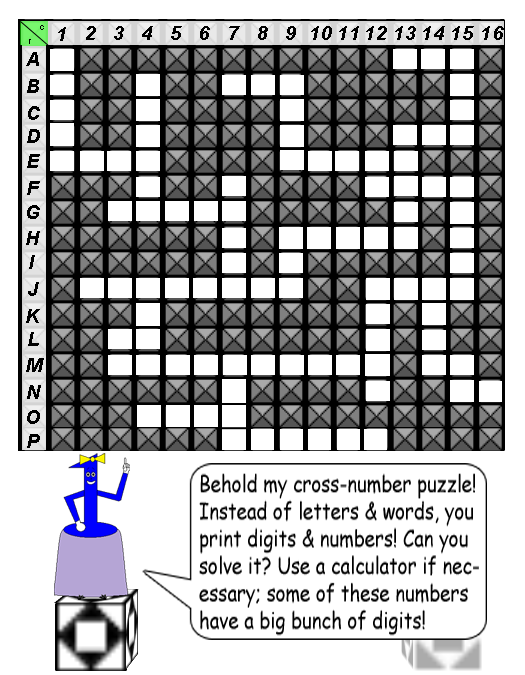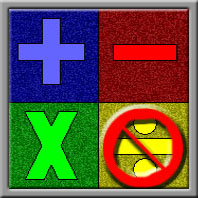# Miss One's Cross-Number Puzzle

#### Difficulty: MediumThe answers to the math problems below are what you have to print in the blank squares. Each blank square represents a single digit in the number of each correct answer to a math problem. Count the number of squares(up-to-down or left-to-right) & you will know how many digits the correct number should have.

As you can see, there are 16 rows & 16 columns; the letters(A-P) stand for the rows, & the numbers(1-16) stand for the columns. The math problems are marked by row letter first, and then column number; for example, a problem marked "A1" means you must print the digit in the number's largest column first in that blank square.

The up-to-down math problems are in the left column, & the left-to-right math problems are in the right column of the table below. You will only get math problems in arithmetic & pre-algebra; division is the only arithmetic operation that has been excluded from this puzzle.P.S.: Remember that pre-algebra rule: "Please Excuse My Dear Aunt Sally!"

### Math Problems:

 Up-to-Down Left-to-Right A1: 14,573 + 50,659 = ? E1: 2^11 = ? B4: 1,684,846 - 1,233,971 = ? G3: 50 × 500 = ? F7: 14,876 + 15,888 = ? J2: 99 × 10^6 + 877,401 = ? H9: 11^2 = ? H9: 11^4 = ? J4: 2^13 + 2^3 = ? L3: 25 × 2 = ? L3: 17 × 3 = ? M3: 10^9 + 10^5 × 5 + 1 = ? M7: 5,000 + 800 + 47 = ? O4: 5,555 - 4,321 = ? J12: 1,099,013 - 1,000,002 = ? P7: 913,555 - 205,124 = ? D13: 1,111 × 9 + 2 = ? J12: 22^3 - 10^3 = ? J14: 13,086 - 8,765 = ? F12: 809 × 5 = ? B9: 99^2 = ? M14: 10 + 7 = ? F15: 151,821 - 94,123 = ? B7: 9^3 = ? M15: 9 × 8 + 7 = ? N15: 100 - 1 = ? A15: 3 × 1,001 = ? E9: 3,125 × 4 + 10 × 2 = ? E12: 10 - 6 + 20 = ? A13: 3^5 = ? D13: 9(125 + 875) + 10^3 + 1 D13: 14^2 - 6^2 + 3 = ?